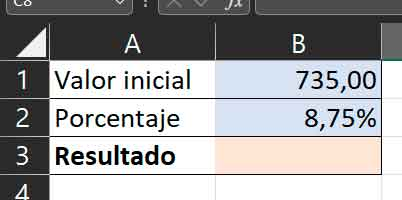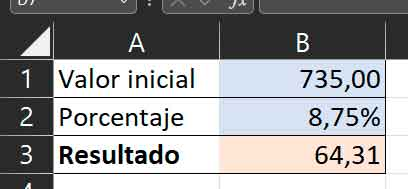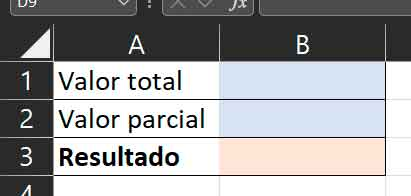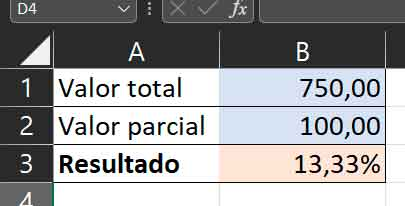# Calculate percentages with Excel

There are many people for whom calculating percentages is an arduous climb. And it is that despite being a simple operation, it is common to see errors in the formulas to calculate them. I won’t name names, but I’ve encountered such errors even on professional websites, which are supposed to help people who have problems with them. I know that may sound like overkill, but I guarantee it’s true.

The chance is that, again, we have the help of Excel, this application that is used for almost everything and that many of us find essential in our daily lives. And yes, it is true that there are many operations that can be performed with a normal calculator, such as the one included in Windows or those that we find on our smartphones (and the calculation of a percentage is one of them), but why not go a little further? We will see three common operations related to percentagesand create a spreadsheet that allows us to calculate them automatically.

#### Calculate the percentage of a number

This is obviously the simplest of these three calculators. What we’re looking for with it is to enter a total and a percentage of it, and have the calculator return the numeric value. Perhaps it’s best understood with an example: if I enter 200 as the total, and 5% as the percentage, the calculator will return a 10, since 10 is 5% of 200.

We’ll start with the visual design of the calculator. In my case, I like to differentiate, by means of colors, in which cells we have to enter data and in which not. We can also block the cells in which the formulas will be included so as not to delete them by mistake, in another practice we will tell you how to do it. In any case, after applying formats and such, my calculator looks like this:As you can deduce, we will need to enter the total in B1 and the percentage in B2. Remember though to apply the proper formats to these cells, i.e. number at B1 and B3 and percentage at B2:All you have to do is go to B3 and write the following formula: “=B1*B2” (without the quotes). And There you go. With this simple formula, you will already have the basic percentage calculator:Remember, yes, change the cell references if you use anything other than the ones shown in the pictures.

#### Calculate the percentage value of one number relative to another

Let’s reverse the situation. Imagine what you want is to fill in two numbers and have the calculator tell you what the percentage value of B is to A. Again with an example, that if you fill in A as 200 and B as 80, the calculator tells you that 80 is 40% of 200.

As for the previous one, create the visual design of this one, in my case it is the following:Of course, in this case you will need to apply the number format to cells B1 and B2, and the percentage to B3. With all that ready, go to B3 and write the formula “=1/B1*B2” (again, of course, without the quotes) and the calculator will be ready:Note that in the example the value of B is less than the value of A, but in reality it can also be used in reverse, for example to calculate the percentage value of a price increase. For this purpose, you can keep in mind that you need to subtract 100 from the result, or you can use this modified version of the formula: “=(1/B1*B2)-1”.#### Break down the share of VAT in a price

Imagine that you paid for a product or service and you only know the final amount you had to pay, but not the amount corresponding to the VAT. The good news is that if you know what rate was applied, it is very easy to calculate it. Remember that in Spain, these are the three types of VAT:

 VAT rate Tax rate General 21.00% Reduced 10.00% Superdiscount 4.00%

And what we want is a calculator to provide the final price we paid and the corresponding VAT rate, and which in response gives us what we paid as a rate and the amount without VAT. In this case, we will therefore use two input fields and two output fields, something like this:In this case, we will apply the currency format to cells B1, B3 and B4 and the percentage format to cell B2. With everything ready, we’ll type the formula “=(B1/(1+B2))*B2” into cell B3, then “=B1-B3” into cell B4. So, when we enter the corresponding values ​​in B1 and B2, we can see the VAT breakdown:As you can see, performing this type of operation is really easy with Excel. And if you want to see more tips about Microsoft spreadsheets, here you can find three more that can be very practical.